### Home > PC > Chapter 1 > Lesson 1.3.2 > Problem1-117

1-117.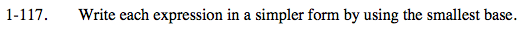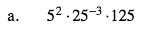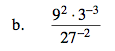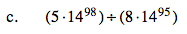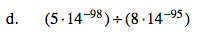$5^2(5^2)^{-3}(5^3)$

Simplify!

$\frac{(3^2)^{2}(3)^{-3}}{(3^3)^{-2}}$

Simplify!

$\frac{5}{8}(14^3)$

$\frac{5}{8}(14^{-3})$